# elektronn3.modules.wsconv module¶

Weight Standartized convolution layers, see https://arxiv.org/abs/1903.10520

class elektronn3.modules.wsconv.FWS(layer, learnable_gain=True, const_eval=False, eps=0.0001)[source]

Bases: torch.nn.

Kind of like weight standardization, but changes weights in place

forward(*args, **kwargs)[source]
standardize_weight()[source]
class elektronn3.modules.wsconv.WSConv1d(*args: Any, **kwargs: Any)[source]

Bases: torch.nn.

Applies a 1D convolution over an input signal composed of several input planes. In the simplest case, the output value of the layer with input size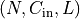and output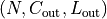can be precisely described as: .. math:

\text{out}(N_i, C_{\text{out}_j}) = \text{bias}(C_{\text{out}_j}) +
\sum_{k = 0}^{C_{in} - 1} \text{weight}(C_{\text{out}_j}, k)
\star \text{input}(N_i, k)


where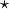is the valid cross-correlation operator,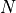is a batch size,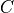denotes a number of channels,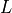is a length of signal sequence. This module supports TensorFloat32. * stride controls the stride for the cross-correlation, a single

number or a one-element tuple.

• padding controls the amount of implicit zero-paddings on both sides for padding number of points.

• dilation controls the spacing between the kernel points; also known as the à trous algorithm. It is harder to describe, but this link has a nice visualization of what dilation does.

• groups controls the connections between inputs and outputs. in_channels and out_channels must both be divisible by groups. For example,

• At groups=1, all inputs are convolved to all outputs.

• At groups=2, the operation becomes equivalent to having two conv layers side by side, each seeing half the input channels, and producing half the output channels, and both subsequently concatenated.

• At groups= in_channels, each input channel is convolved with its own set of filters, of size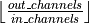.

Note

Depending of the size of your kernel, several (of the last) columns of the input might be lost, because it is a valid cross-correlation, and not a full cross-correlation. It is up to the user to add proper padding.

Note

When groups == in_channels and out_channels == K * in_channels, where K is a positive integer, this operation is also termed in literature as depthwise convolution. In other words, for an input of size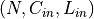, a depthwise convolution with a depthwise multiplier K, can be constructed by arguments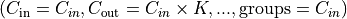.

Note

In some circumstances when using the CUDA backend with CuDNN, this operator may select a nondeterministic algorithm to increase performance. If this is undesirable, you can try to make the operation deterministic (potentially at a performance cost) by setting torch.backends.cudnn.deterministic = True. Please see the notes on /notes/randomness for background.

Parameters
• in_channels (int) – Number of channels in the input image

• out_channels (int) – Number of channels produced by the convolution

• kernel_size (int or tuple) – Size of the convolving kernel

• stride (int or tuple, optional) – Stride of the convolution. Default: 1

• padding (int or tuple, optional) – Zero-padding added to both sides of the input. Default: 0

• padding_mode (string, optional) – 'zeros', 'reflect', 'replicate' or 'circular'. Default: 'zeros'

• dilation (int or tuple, optional) – Spacing between kernel elements. Default: 1

• groups (int, optional) – Number of blocked connections from input channels to output channels. Default: 1

• bias (bool, optional) – If True, adds a learnable bias to the output. Default: True

Shape:
• Input:• Output: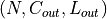where .. math:

L_{out} = \left\lfloor\frac{L_{in} + 2 \times \text{padding} - \text{dilation}
\times (\text{kernel\_size} - 1) - 1}{\text{stride}} + 1\right\rfloor

weight

the learnable weights of the module of shape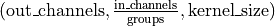. The values of these weights are sampled from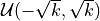where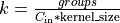Type

Tensor

bias

the learnable bias of the module of shape (out_channels). If bias is True, then the values of these weights are sampled fromwhereType

Tensor

Examples::
>>> m = nn.Conv1d(16, 33, 3, stride=2)
>>> input = torch.randn(20, 16, 50)
>>> output = m(input)

forward(input, eps=0.0001)[source]
standardize_weight(eps)[source]
class elektronn3.modules.wsconv.WSConv2d(*args: Any, **kwargs: Any)[source]

Bases: torch.nn.

Applies a 2D convolution over an input signal composed of several input

planes after weight normalization/standardization. Reference: https://github.com/deepmind/deepmind-research/blob/master/nfnets/base.py#L121 In the simplest case, the output value of the layer with input size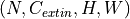and output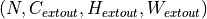can be precisely described as: .. math:

        ext{out}(N_i, C_{       ext{out}_j}) =  ext{bias}(C_{   ext{out}_j}) +
\sum_{k = 0}^{C_{       ext{in}} - 1}   ext{weight}(C_{ ext{out}_j}, k) \star   ext{input}(N_i, k)


whereis the valid 2D cross-correlation operator,is a batch size,denotes a number of channels,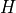is a height of input planes in pixels, and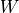is width in pixels. This module supports TensorFloat32. * stride controls the stride for the cross-correlation, a single

number or a tuple.

• padding controls the amount of implicit zero-paddings on both sides for padding number of points for each dimension.

• dilation controls the spacing between the kernel points; also known as the à trous algorithm. It is harder to describe, but this link has a nice visualization of what dilation does.

• groups controls the connections between inputs and outputs. in_channels and out_channels must both be divisible by groups. For example,

• At groups=1, all inputs are convolved to all outputs.

• At groups=2, the operation becomes equivalent to having two conv layers side by side, each seeing half the input channels, and producing half the output channels, and both subsequently concatenated.

• At groups= in_channels, each input channel is convolved with its own set of filters, of size: :math:leftlfloor

rac{out_channels}{in_channels} ight floor.

The parameters kernel_size, stride, padding, dilation can either be:
• a single int – in which case the same value is used for the height and width dimension

• a tuple of two ints – in which case, the first int is used for the height dimension, and the second int for the width dimension

Note:

Depending of the size of your kernel, several (of the last) columns of the input might be lost, because it is a valid cross-correlation, and not a full cross-correlation. It is up to the user to add proper padding.

Note:

When groups == in_channels and out_channels == K * in_channels, where K is a positive integer, this operation is also termed in literature as depthwise convolution. In other words, for an input of size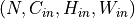, a depthwise convolution with a depthwise multiplier K, can be constructed by arguments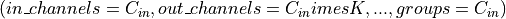.

Note:

In some circumstances when using the CUDA backend with CuDNN, this operator may select a nondeterministic algorithm to increase performance. If this is undesirable, you can try to make the operation deterministic (potentially at a performance cost) by setting torch.backends.cudnn.deterministic = True. Please see the notes on /notes/randomness for background.

Args:

in_channels (int): Number of channels in the input image out_channels (int): Number of channels produced by the convolution kernel_size (int or tuple): Size of the convolving kernel stride (int or tuple, optional): Stride of the convolution. Default: 1 padding (int or tuple, optional): Zero-padding added to both sides of

the input. Default: 0

padding_mode (string, optional): 'zeros', 'reflect',

'replicate' or 'circular'. Default: 'zeros'

dilation (int or tuple, optional): Spacing between kernel elements. Default: 1 groups (int, optional): Number of blocked connections from input

channels to output channels. Default: 1

bias (bool, optional): If True, adds a learnable bias to the

output. Default: True

Shape:
• Input:• Output: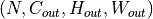where .. math:

H_{out} = \left\lfloor

rac{H_{in} + 2 imes ext{padding} - ext{dilation}

imes ( ext{kernel_size} - 1) - 1}{ ext{stride}} + 1

ight floor

System Message: WARNING/2 (W_{out} = \left\lfloor )

latex exited with error [stdout] This is pdfTeX, Version 3.14159265-2.6-1.40.18 (TeX Live 2017/Debian) (preloaded format=latex) restricted \write18 enabled. entering extended mode (./math.tex LaTeX2e <2017-04-15> Babel <3.18> and hyphenation patterns for 84 language(s) loaded. (/usr/share/texlive/texmf-dist/tex/latex/base/article.cls Document Class: article 2014/09/29 v1.4h Standard LaTeX document class (/usr/share/texlive/texmf-dist/tex/latex/base/size12.clo)) (/usr/share/texlive/texmf-dist/tex/latex/base/inputenc.sty (/usr/share/texlive/texmf-dist/tex/latex/base/utf8.def (/usr/share/texlive/texmf-dist/tex/latex/base/t1enc.dfu) (/usr/share/texlive/texmf-dist/tex/latex/base/ot1enc.dfu) (/usr/share/texlive/texmf-dist/tex/latex/base/omsenc.dfu))) (/usr/share/texlive/texmf-dist/tex/latex/amsmath/amsmath.sty For additional information on amsmath, use the ?' option. (/usr/share/texlive/texmf-dist/tex/latex/amsmath/amstext.sty (/usr/share/texlive/texmf-dist/tex/latex/amsmath/amsgen.sty)) (/usr/share/texlive/texmf-dist/tex/latex/amsmath/amsbsy.sty) (/usr/share/texlive/texmf-dist/tex/latex/amsmath/amsopn.sty)) (/usr/share/texlive/texmf-dist/tex/latex/amscls/amsthm.sty) (/usr/share/texlive/texmf-dist/tex/latex/amsfonts/amssymb.sty (/usr/share/texlive/texmf-dist/tex/latex/amsfonts/amsfonts.sty)) (/usr/share/texlive/texmf-dist/tex/latex/anyfontsize/anyfontsize.sty) (/usr/share/texlive/texmf-dist/tex/latex/tools/bm.sty) (./math.aux) (/usr/share/texlive/texmf-dist/tex/latex/amsfonts/umsa.fd) (/usr/share/texlive/texmf-dist/tex/latex/amsfonts/umsb.fd) ! Extra }, or forgotten \right. <template> } $\endtemplate l.14 ...in{split}W_{out} = \left\lfloor\end{split} ! Missing \right. inserted. <inserted text> \right . l.14 ...in{split}W_{out} = \left\lfloor\end{split} ! Missing } inserted. <inserted text> } l.14 ...in{split}W_{out} = \left\lfloor\end{split} ! Extra }, or forgotten \right. <template> }$\endtemplate l.14 ...in{split}W_{out} = \left\lfloor\end{split} ! Missing \right. inserted. <inserted text> \right . l.14 ...in{split}W_{out} = \left\lfloor\end{split} ! Missing } inserted. <inserted text> } l.14 ...in{split}W_{out} = \left\lfloor\end{split}  (./math.aux) ) (see the transcript file for additional information) Output written on math.dvi (1 page, 392 bytes). Transcript written on math.log.

rac{W_{in} + 2 imes ext{padding} - ext{dilation}

imes ( ext{kernel_size} - 1) - 1}{ ext{stride}} + 1

ight floor

Attributes:
weight (Tensor): the learnable weights of the module of shape

:math:( ext{out_channels},

rac{ ext{in_channels}}{ ext{groups}},

:math: ext{kernel_size}, ext{kernel_size}). The values of these weights are sampled fromwhere :math:k =

rac{groups}{C_ ext{in} * prod_{i=0}^{1} ext{kernel_size}[i]}
bias (Tensor): the learnable bias of the module of shape

(out_channels). If bias is True, then the values of these weights are sampled fromwhere :math:k =

rac{groups}{C_ ext{in} * prod_{i=0}^{1} ext{kernel_size}[i]}
Examples:
>>> # With square kernels and equal stride
>>> m = WSConv2d(16, 33, 3, stride=2)
>>> # non-square kernels and unequal stride and with padding
>>> m = WSConv2d(16, 33, (3, 5), stride=(2, 1), padding=(4, 2))
>>> # non-square kernels and unequal stride and with padding and dilation
>>> m = WSConv2d(16, 33, (3, 5), stride=(2, 1), padding=(4, 2), dilation=(3, 1))
>>> input = torch.randn(20, 16, 50, 100)
>>> output = m(input)

forward(input, eps=0.0001)[source]
standardize_weight(eps)[source]
class elektronn3.modules.wsconv.WSConv3d(*args: Any, **kwargs: Any)[source]

Bases: torch.nn.

forward(input, eps=0.0001)[source]
standardize_weight(eps)[source]

Bases: torch.nn.

Applies a 2D transposed convolution operator over an input image

composed of several input planes after weight normalization/standardization. This module can be seen as the gradient of Conv2d with respect to its input. It is also known as a fractionally-strided convolution or a deconvolution (although it is not an actual deconvolution operation). This module supports TensorFloat32. * stride controls the stride for the cross-correlation. * padding controls the amount of implicit zero-paddings on both

sides for dilation * (kernel_size - 1) - padding number of points. See note below for details.

• output_padding controls the additional size added to one side of the output shape. See note below for details.

• dilation controls the spacing between the kernel points; also known as the à trous algorithm. It is harder to describe, but this link has a nice visualization of what dilation does.

• groups controls the connections between inputs and outputs. in_channels and out_channels must both be divisible by groups. For example,

• At groups=1, all inputs are convolved to all outputs.

• At groups=2, the operation becomes equivalent to having two conv layers side by side, each seeing half the input channels, and producing half the output channels, and both subsequently concatenated.

• At groups= in_channels, each input channel is convolved with its own set of filters (of size :math:leftlfloor

rac{out_channels}{in_channels} ight floor).

The parameters kernel_size, stride, padding, output_padding can either be:

• a single int – in which case the same value is used for the height and width dimensions

• a tuple of two ints – in which case, the first int is used for the height dimension, and the second int for the width dimension

Note

Depending of the size of your kernel, several (of the last) columns of the input might be lost, because it is a valid cross-correlation, and not a full cross-correlation. It is up to the user to add proper padding.

Note:

The padding argument effectively adds dilation * (kernel_size - 1) - padding amount of zero padding to both sizes of the input. This is set so that when a Conv2d and a ConvTranspose2d are initialized with same parameters, they are inverses of each other in regard to the input and output shapes. However, when stride > 1, Conv2d maps multiple input shapes to the same output shape. output_padding is provided to resolve this ambiguity by effectively increasing the calculated output shape on one side. Note that output_padding is only used to find output shape, but does not actually add zero-padding to output.

Note:

In some circumstances when using the CUDA backend with CuDNN, this operator may select a nondeterministic algorithm to increase performance. If this is undesirable, you can try to make the operation deterministic (potentially at a performance cost) by setting torch.backends.cudnn.deterministic = True. Please see the notes on /notes/randomness for background.

Args:

in_channels (int): Number of channels in the input image out_channels (int): Number of channels produced by the convolution kernel_size (int or tuple): Size of the convolving kernel stride (int or tuple, optional): Stride of the convolution. Default: 1 padding (int or tuple, optional): dilation * (kernel_size - 1) - padding zero-padding

will be added to both sides of each dimension in the input. Default: 0

of each dimension in the output shape. Default: 0

groups (int, optional): Number of blocked connections from input channels to output channels. Default: 1 bias (bool, optional): If True, adds a learnable bias to the output. Default: True dilation (int or tuple, optional): Spacing between kernel elements. Default: 1

Shape:
• Input:• Output:where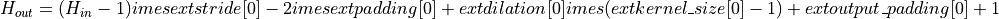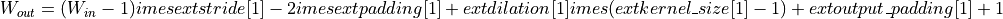Attributes:
weight (Tensor): the learnable weights of the module of shape

:math:( ext{in_channels},

rac{ ext{out_channels}}{ ext{groups}},

:math: ext{kernel_size}, ext{kernel_size}). The values of these weights are sampled fromwhere :math:k =

rac{groups}{C_ ext{out} * prod_{i=0}^{1} ext{kernel_size}[i]}
bias (Tensor): the learnable bias of the module of shape (out_channels)

If bias is True, then the values of these weights are sampled fromwhere :math:k =

rac{groups}{C_ ext{out} * prod_{i=0}^{1} ext{kernel_size}[i]}
Examples::
>>> # With square kernels and equal stride
>>> m = WSConvTranspose2d(16, 33, 3, stride=2)
>>> # non-square kernels and unequal stride and with padding
>>> m = WSConvTranspose2d(16, 33, (3, 5), stride=(2, 1), padding=(4, 2))
>>> input = torch.randn(20, 16, 50, 100)
>>> output = m(input)
>>> # exact output size can be also specified as an argument
>>> input = torch.randn(1, 16, 12, 12)
>>> downsample = WSConv2d(16, 16, 3, stride=2, padding=1)
>>> upsample = WSConvTranspose2d(16, 16, 3, stride=2, padding=1)
>>> h = downsample(input)
>>> h.size()
torch.Size([1, 16, 6, 6])
>>> output = upsample(h, output_size=input.size())
>>> output.size()
torch.Size([1, 16, 12, 12])

forward(input, output_size=None, eps=0.0001)[source]
Return type

Tensor

standardize_weight(eps)[source]
Bases: torch.nn.
Tensor`